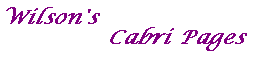#### Proof of Theorem 3

Theorem 3 La Hire's Theorem
Suppose that C: xTMx=0 is a non-degenerate conic and that U and V are any points.
Then U lies on the polar of V if and only if V lies on the polar of U.

Proof
Suppose that U=[u] and that V=[v].
Then each condition is clearly equivalent to uTMv=0.